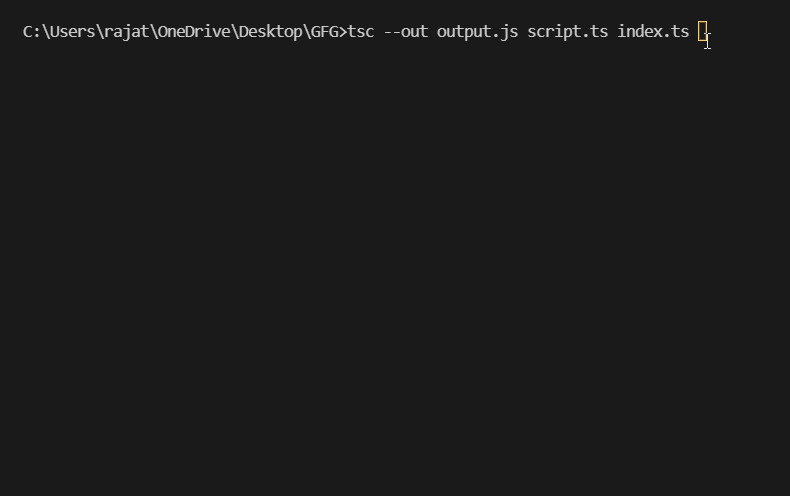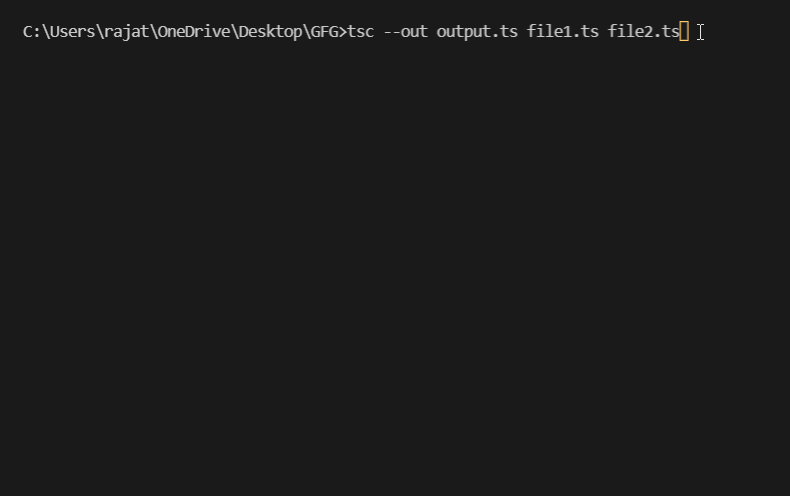# How to compile multiple Typescript files into a single file ?

• Last Updated : 09 Mar, 2022

In this article, we will learn how to compile multiple Typescript files into a single file. There are two approaches that can be followed here:

Approach 1: Compiling multiple Typescript files into a single JavaScript file. We simply use the following syntax:

Syntax:

tsc –out outputFile.js typeScriptFile1.ts typeScriptFile2.ts … typeScriptFilen.ts

Explanation:

• tsc: It stands for TypeScript compiler which is used to invoke the compiler in order to compile the TypeScript files.
• –out: It is a CLI (Command Line Interface) command which concatenates the TypeScript files and emits the output to a single JS file.
• outputFile.js: The JavaScript file which holds the result of the compilation of the TypeScript files.
• typeScriptFile1, typeScriptFile2 … typeScriptFilen: The TypeScript files to be compiled.

Example: Here, three TypeScript files with the names script.ts, index.ts and app.ts are compiled to a single JavaScript file output.js. Subsequently, the JavaScript file is executed by using the following CLI command:

`node output.js`

## script.ts

 `const myArr = [1, 2, 3, 4, 5]; ` ` `  `console.log(``"CONTENTS OF FILE 1"``); ` ` `  `for` `(let i = 0; i < myArr.length; i++) { ` `  ``console.log(myArr[i]); ` `}`

## index.ts

 `const GFG = [``"Geeks"``, ``"For"``, ``"Geeks"``]; ` ` `  `console.log(``"\nCONTENTS OF FILE 2"``); ` ` `  `for` `(let i = 0; i < GFG.length; i++) { ` `  ``console.log(GFG[i]); ` `}`

## app.ts

 `const geeks = [``true``, ``false``, 2.5, 5]; ` ` `  `console.log(``"\nCONTENTS OF FILE 3"``); ` ` `  `for` `(let i = 0; i < geeks.length; i++) { ` `  ``console.log(geeks[i]); ` `}`

Generated output JavaScript file “output.js”

## output.js

 `var` `myArr = [1, 2, 3, 4, 5]; ` ` `  `console.log(``"CONTENTS OF FILE 1"``); ` ` `  `for` `(``var` `i = 0; i < myArr.length; i++) { ` `    ``console.log(myArr[i]); ` `} ` ` `  `var` `GFG = [``"Geeks"``, ``"For"``, ``"Geeks"``]; ` ` `  `console.log(``"\nCONTENTS OF FILE 2"``); ` ` `  `for` `(``var` `i = 0; i < GFG.length; i++) { ` `    ``console.log(GFG[i]); ` `} ` ` `  `var` `geeks = [``true``, ``false``, 2.5, 5]; ` ` `  `console.log(``"\nCONTENTS OF FILE 3"``); ` ` `  `for` `(``var` `i = 0; i < geeks.length; i++) { ` `    ``console.log(geeks[i]); ` `}`

Output:Approach 2: Compiling multiple Typescript files into a single TypeScript file. We use the following syntax:

Syntax:

tsc –out outputFile.ts typeScriptFile1.ts typeScriptFile2.ts … typeScriptFilen.ts

Explanation:

• tsc: It stands for TypeScript compiler which is used to invoke the compiler in order to compile the TypeScript files.
• –out: It is a CLI (Command Line Interface) command which concatenates the TypeScript files and emits the output to a single TypeScript file
• outputFile.ts: The TypeScript file which holds the result of the compilation of the TypeScript files
• typeScriptFile1, typeScriptFile2 … typeScriptFilen: The TypeScript files to be compiled.

Example: Here, three TypeScript files with the names file1.ts, file2.ts and file3.ts are compiled to a single TypeScript file output.ts. After that, the resultant TypeScript file is compiled to a JavaScript file output.js and then the JavaScript file is executed by using the following CLI commands:

```tsc output.ts
node output.js```

## file1.ts

 `let i = 1; ` `console.log(``"CONTENTS OF FILE 1"``); ` `while` `(i <= 5) { ` `  ``console.log(i); ` `  ``i++; ` `}`

## file2.ts

 `console.log(``"\nCONTENTS OF FILE 2"``); ` `console.log(``"GeeksForGeeks is a computer science portal for geeks."``);`

## file3.ts

 `const geeks = [2, 4, 6, 8]; ` `console.log(``"\nCONTENTS OF FILE 3"``); ` `for` `(let i = 0; i < geeks.length; i++) { ` `  ``console.log(geeks[i]); ` `}`

Generated output TypeScript file “output.ts”

## output.ts

 `var` `i = 1; ` `console.log(``"CONTENTS OF FILE 1"``); ` `while` `(i <= 5) { ` `    ``console.log(i); ` `    ``i++; ` `} ` `console.log(``"\nCONTENTS OF FILE 2"``); ` `console.log(``"GeeksForGeeks is a computer science portal for geeks."``); ` `var` `geeks = [2, 4, 6, 8]; ` `console.log(``"\nCONTENTS OF FILE 3"``); ` `for` `(``var` `i_1 = 0; i_1 < geeks.length; i_1++) { ` `    ``console.log(geeks[i_1]); ` `}`

Generated output JavaScript file “output.js”

## output.js

 `var` `i = 1; ` `console.log(``"CONTENTS OF FILE 1"``); ` `while` `(i <= 5) { ` `    ``console.log(i); ` `    ``i++; ` `} ` `console.log(``"\nCONTENTS OF FILE 2"``); ` `console.log(``"GeeksForGeeks is a computer science portal for geeks."``); ` `var` `geeks = [2, 4, 6, 8]; ` `console.log(``"\nCONTENTS OF FILE 3"``); ` `for` `(``var` `i_1 = 0; i_1 < geeks.length; i_1++) { ` `    ``console.log(geeks[i_1]); ` `}`

Output:My Personal Notes arrow_drop_up
Recommended Articles
Page :